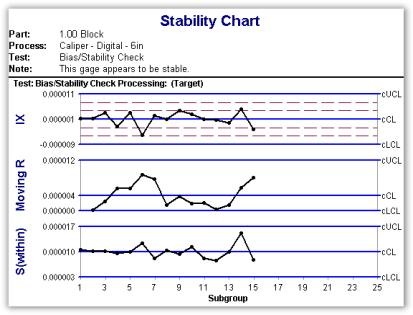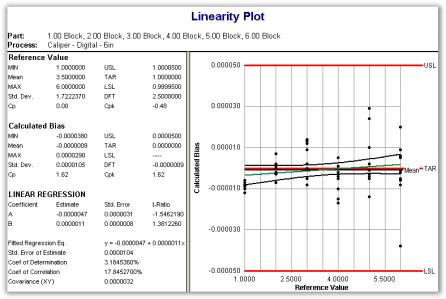# Using InfinityQS to Measure Bias, Stability and Linearity

Like a process, a measurement system is influenced by many sources of variation. These influences may have adverse affects. A measurement system exhibits variation in the mean and variability of the test results. Gage studies are designed to detect when a measurement system's variation has reached unsuitable levels. The most common type of gage study is call a GR&R, for gage repeatability and reproducibility. A GR&R study determines the level of a gage's variability. Three other studies called BiasStability andLinearity are used to detect a gage's mean, or location.

### Bias

Bias is the difference between a reference value and the measurement system's observed value. To test for bias, one measures a reference several times and compares the mean of the measurements to the reference value.

Bias = Observed Value - Reference Value

Part = Reference
Process = Gage Device
Test #1 = Bias Check [manual or gage input]
Specification Limit = the reference as the target and a USL and LSL to represent the maximum allowable bias.

Set up a data entry configuration with a subgroup size of 10. The data can be plotted as either a control chart or a box plot with the values processed as deviation from target. Each time a bias check is performed, a new plot point is generated on the control chart. When creating the box plot, set the major category to subgroup. With this setting, each new bias check will generate a new box.

### Stability

Stability is study of a series of bias checks over a period of time. A control chart is used to analyze stability. There is no stability number.How to Set Up an InfinityQS Project to Check Stability:

Using the setup described for bias checks, stability is analyzed by looking at a series of bias plot points on a control chart. It is recommended that a Target 3D chart be used. The plot points on the top chart represent stability in the gage's mean. The middle chart (moving-range) represents the gage's stability from one time to the next. The bottom chart (range-within) represents the stability within a bias check. These two range charts are actually a time-ordered look at a gage's reproducibility (MR) and repeatability (R within).

The same data entry configuration can be used for both the bias and stability checks.

### Linearity

A linearity study is performed by gathering the bias values from multiple references (typically gage blocks) that represent the gage's full operating range. Linearity studies are preformed to ensure that the gage's bias values are acceptable throughout the gage's operating range.How to Set Up an InfinityQS Project to Check Linearity:

Since linearity studies require calculated fields, a different data entry configuration as well as different test items are required. However, both data entry configurations can reside in the same project. Below are the naming conventions used in this example:

Part = Reference
Process = Gage
Test #1 = Reference Value [set this to the TAR ( ) calculated field]
Test #2 = Linearity Check [manual or gage input]
Test #3 = Calculated Bias [calculated field: Test #2 - Test #1]

With this data entry configuration, Test #1 will automatically fill with the part's (reference) target value, the appraiser inputs the measurements under Test #2 and the software automatically calculates the bias in Test #3. The chart to use is a Scatter Plot. Set the x-axis to Reference Value and the y-axis to Calculated Bias. Enable the best fit (regression) line and specify the axis grid settings appropriately. The result is a standard linearity plot with all the flexibility of an InfinityQS project.

Within the data entry configuration, set the part (reference) as re-select on add. With the first subgroup, select the smallest reference and keep adding subgroups until all references have been measured.

### Summary

As demonstrated in this example, the built-in tools of InfinityQS SPC support the needs of those customers needing to track their measurement systems' bias, stability and linearity. Bias and stability are tracked using target charts and/or box plots while linearity is illustrated using the scatter plot. This example can be viewed in the Demo Projects folder.

Need help?
​For further information contact GetInTouch@infinityqs.com
Toll Free: 1.800.772.7978

### Take the first step from quality to excellence

Take the Next Steps